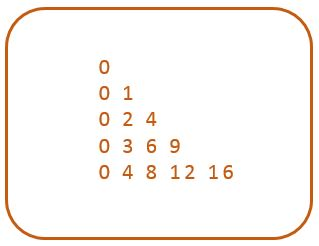# C program to print right triangle pyramid pattern of multiplication tables

• Write a program in C to print right triangle pattern of multiplication table.
• How to draw a pyramid pattern multiplication table using for loop in C.
Here is a sample right triangle pattern of multiplication table.

### MULTIPLICATION TABLE TRIANGLE#### Required Knowledge

Algorithm to print triangle pattern of multiplication table
This program is similar to right triangle star pattern.
• Take the number of rows(N) of right triangle as input from user using scanf function.
• Number of integers in Kth row is always K.
• In any row R, we will print multiplication table of R-1. Multiplication factor will start from 0 and continue till R-1. For Example, 4th row will print multiplication table of 3 till 4 terms and from factor 0 to 3.

## C program to print multiplication table pyramid pattern

```#include<stdio.h>

int main() {
int i, j, rows;
int count = 1;

printf("Enter the number of rows\n");
scanf("%d", &rows);

for (i = 0; i < rows; i++) {
for (j = 0; j <= i; j++) {
printf("%d ", i*j);
}
printf("\n");
}
return(0);
}
```
Output
```Enter the number of rows
6
0
0 1
0 2 4
0 3 6 9
0 4 8 12 16
0 5 10 15 20 25
```

Related Topics
 C program prime number triangle pattern C program binary triangle pattern C program right triangle star pattern C program inverted right triangle pattern C program mirrored right triangle star pattern C program reversed right triangle star pattern C program heart shape star pattern C program reversed pyramid star pattern C program diamond star pattern List of all C pattern programs